## Time Frequency Analysis

Time-frequency analysis identifies the time at which various signal frequencies are present, usually by calculating a spectrum at regular intervals of time.

### Sonogram

Also called the “Short-time Fourier Transform”, a sonogram is a two-dimensional image created by computing the Fourier spectra using a sliding temporal window: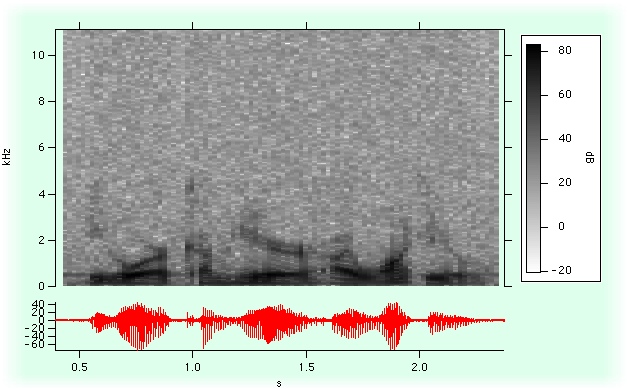By adjusting the width of the window you can determine the time resolution of the resulting spectra: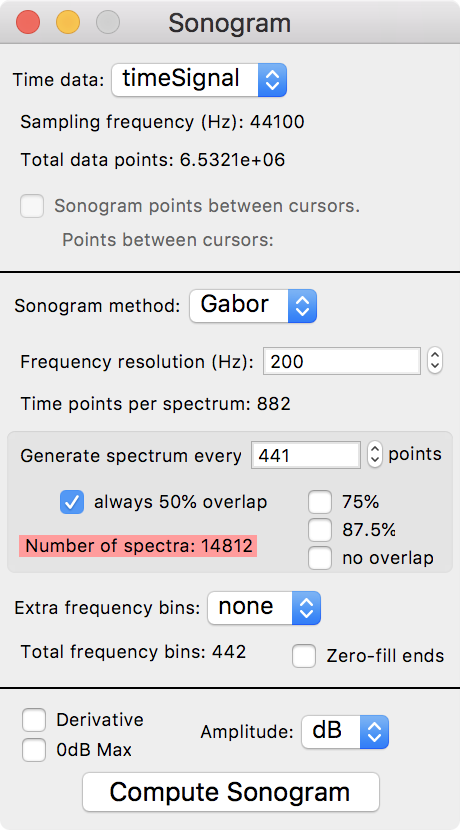### Wigner Transform

The Wigner transform (also known as the Wigner Distribution Function or WDF) maps a 1D time signal U(t) into a 2D time-frequency representation. Conceptually, the WDF is analogous to a musical score where the time axis is horizontal and the frequencies (notes) are plotted on a vertical axis. The WDF is defined by the equation: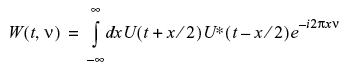Note that the WDF W(t, ν) is real (this can be seen from the fact that it is a Fourier transform of an Hermitian quantity). The WDF is also a 2D Fourier transform of the Ambiguity function. The localized spectrum can be derived from the WDF by integrating it over a finite area ∂t∂ν. Using Gaussian weight functions in both t and ν, and choosing the minimum uncertainty condition ∂t∂ν=1, we obtain an estimate for the local spectrum: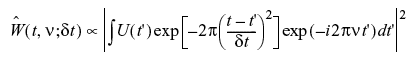To illustrate an application of the WignerTransform operation, consider a two-frequency signal, its Wigner Transform, and its periodogram:

• ```Make/N=500 signal
signal[0,350]=sin(2*pi*x*50/500)
signal[250,]+=sin(2*pi*x*100/500)
WignerTransform /Gaus=100 signal
DSPPeriodogram signal // spectrum for comparison
```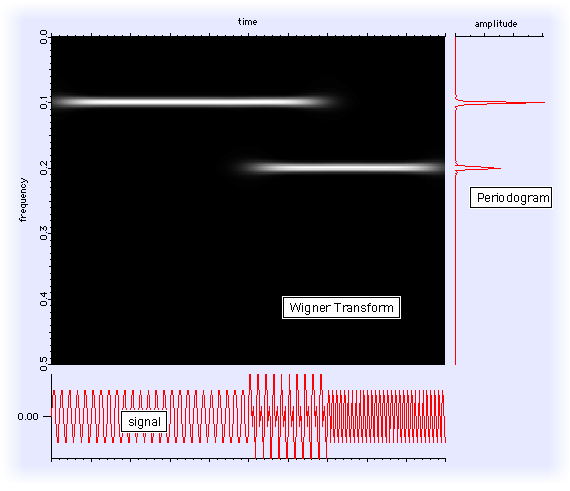### Continuous Wavelet Transform

The Continuous Wavelet Transform (CWT) is a time-frequency representation of signals that graphically has a superficial similarity to the Wigner transform.

A wavelet transform is a convolution of a signal s(t) with a set of functions which are generated by translations and dilations of a main function. The main function is known as the mother wavelet and the translated or dilated functions are called wavelets. Mathematically, the CWT is given by: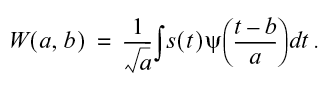Here b is the time translation and a is the dilation of the wavelet.

From a computational point of view it is natural to use the FFT to compute the convolution which suggests that the results are dependent on proper sampling of s(t).

When the mother wavelet is complex, the CWT is also a complex valued function. Otherwise the CWT is real. The squared magnitude of the CWT |W(a,b)|² is equivalent to the power spectrum so that a typical display (image) of the CWT is a representation of the power spectrum as a function of time offset b. One should note however that the precise form of the CWT depends on the choice of mother wavelet y and therefore the extent of the equivalency between the squared magnitude of the CWT and the power spectrum is application dependent.

The CWT operation is implemented using both the FFT and the discrete sum approach. You can use either one to get a representation of the effective wavelet using a delta function as an input.

### CWT Example

• ```Make/O/N=1000 signal=sin(2*pi*x*50/1000)	// test signal is simple sine wave
CWT/OUT=4/SMP2=1/R2={1,1,40}/WBI1=MorletC/FSCL/M=1/ENDM=2 signal
```

The two-dimensional M_CWT result displayed as an image: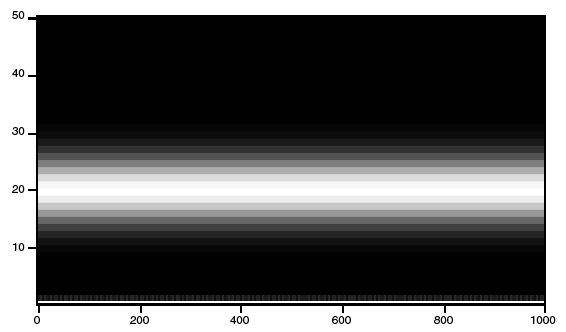### Discrete Wavelet Transform

The Discrete Wavelet Transform (DWT) is similar to the Fourier transform in that it is a decomposition of a signal in terms of a basis set of functions. In Fourier transforms the basis set consists of sines and cosines and the expansion has a single parameter. In wavelet transform the expansion has two parameters and the functions (wavelets) are generated from a single "mother" wavelet using dilation and offsets corresponding to the two parameters.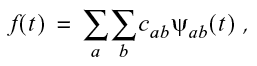where the two-parameter expansion coefficients are given by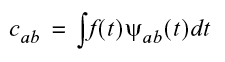and the wavelets obey the condition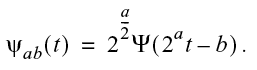Here Ψ is the mother wavelet, a is the dilation parameter and b is the offset parameter.

The two parameter representation can complicate things quickly as one goes from 1D signal to higher dimensions. In addition, because the number of coefficients in each scale varies as a power of 2, the DWT of a 1D signal is not conveniently represented as a 2D image (as is the case with the CWT). It is therefore customary to "pack" the results of the transform so that they have the same dimensionality of the input. For example, if the input is a 1D wave of 128 (=27) points, there are 7-1=6 significant scales arranged as follows: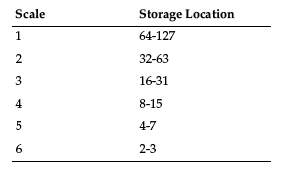An interesting consequence of the definition of the DWT is that you can find out the shape of the wavelet by inverse transforming a suitable form of a delta function. For example:

• ```Make/N=1024 delta=0
delta=1
DWT/I delta
Display W_DWT // Daubechies 4 coefficient wavelet
```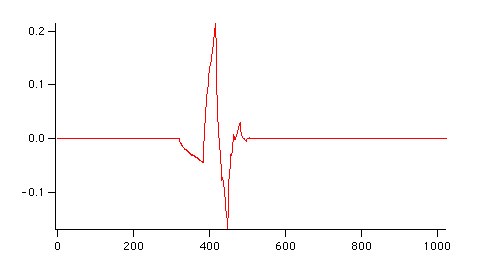The important thing about the DWT basis function is that it is exactly zero outside of a certain duration. This is different from the Fourier transform whose basis function is the un-ending sine wave.

This difference is the foundation for one of the more popular applications of the DWT: "de-noising".

#### Removing Noise with the DWT

“De-noising” a signal with the DWT involves three steps:

1. Transform the input signal with the DWT.
2. Zero all transform coefficients whose magnitude falls below some percentage of the maximum magnitude of the transform.
3. Inverse DWT.

The Wavelet Demo Example experiment demonstrates this technique: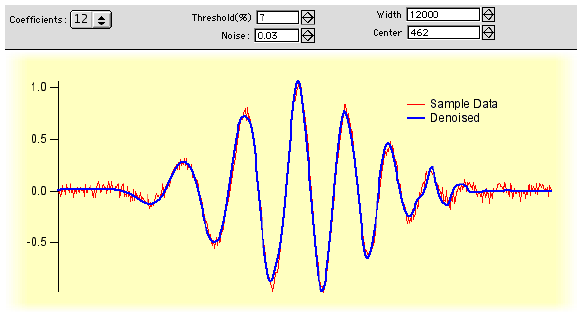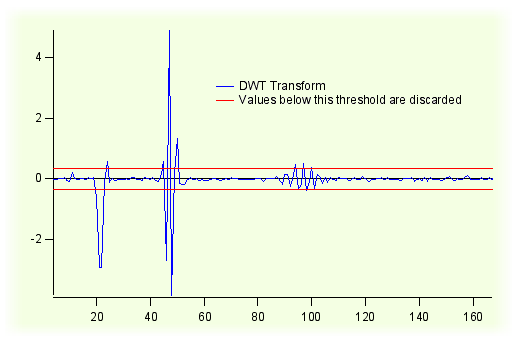Igor’s DWT operation performs de-noising when the /D flag is specified.

### References

• Wigner, E. P., On the quantum correction for thermo-dynamic equilibrium, Physics Review, 40, 749-759, 1932.
• Bartelt, H.O., K.-H. Brenner, and A.W. Lohman, The wigner distribution function and its optical production, Optics Communications, 32, 32-38, 1980.
• Torrence, C., and G.P. Compo, A Practical Guide to Wavelet Analysis, Bulletin of the American Meteorological Society, 79, 61-78, 1998.

The Torrence and Compo journal article is also online at: http://paos.colorado.edu/research/wavelets/.ForumSupportGallery